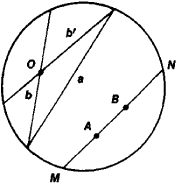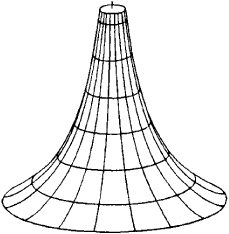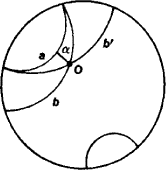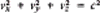# Lobachevskian Geometry

The following article is from The Great Soviet Encyclopedia (1979). It might be outdated or ideologically biased.

## Lobachevskian Geometry

a geometric theory based on the same fundamental premises as ordinary Euclidean geometry, with the exception of the parallel postulate, which is replaced by Lobachevskii’s parallel postulate. Euclid’s parallel postulate states that through a point not on a given line there passes one and only one line lying in the same plane and not intersecting the given line. In Lobachevskian geometry the following axiom is used instead of Euclid’s parallel postulate: through a point not on a given line there pass at least two lines lying in the same plane and not intersecting the given line. It would seem that this axiom contradicts customary concepts. Nevertheless, both this axiom and the entirety of Lobachevskian geometry have quite real meaning (see below).

Lobachevskian geometry was created and developed by N. I. Lobachevskii, who first presented it in 1826. Lobachevskian geometry is called non-Euclidean geometry, although the term “non-Euclidean” is generally assigned a broader meaning that includes theories that arose soon after Lobachevskian geometry and were also based on changing the fundamental premises of Euclidean geometry. Lobachevskian geometry is a hyperbolic non-Euclidean geometry, in contrast to Riemann’s elliptic geometry.

Lobachevskian geometry is a theory rich in content and with applications both in mathematics and physics. Its historical significance is that Lobachevskii by constructing it proved the existence of a geometry differing from Euclidean geometry. This heralded a new era in the development of geometry and of mathematics in general. One modern way of defining Lobachevskian geometry is to say that it is the geometry of a disk, that is, the interior of a circle (Figure 1), in an ordinary (Euclidean) plane,Figure 1

with suitable changes of terminology. Specifically, the disk is called a plane and its points are called points in the “plane,” and its chords (such as a, b, b ‘, MN) are called lines; the transformations of the disk that send chords to chords are called motions. Accordingly, two figures in the disk are said to be congruent (equal) if there is a motion that sends one of these figures to the other. Any geometric fact described in this language proves to be a theorem or axiom of Lobachevskian geometry. In other words, any assertion of plane Lobachevskian geometry is an assertion of Euclidean geometry that pertains to figures in the disk but is expressed in the terms just introduced. It is clear that Euclid’s parallel postulate is not valid in our geometry, since through a point O not on a given chord a (that is, “line”) there pass infinitely many chords (“lines”) that do not intersect it (such as b and b’). Similarly, solid Lobachevskian geometry can be defined as the geometry of a Euclidean spheroid, that is, the interior of a Euclidean sphere, with suitable changes of terminology. Specifically, “lines” are chords, “planes” are plane sections of the spheroid, and “congruent” figures are figures that can be mapped to each other by transformations of the spheroid that send chords to chords. Thus, Lobachevskian geometry is meaningful and just as consistent as Euclidean geometry. The description of the same facts in different terms or, conversely, the description of different facts in the same terms is a characteristic feature of mathematics. This is clearly evident, for example, when a given curve is defined in different coordinate systems by different equations, or conversely, when a given equation represents different curves in different coordinate systems.

Origin. Lobachevskian geometry stems from the problem of the nature of the parallel postulate, which is also known as Euclid’s fifth postulate (this is the number in Euclid’s Elements of the assertion equivalent to the parallel postulate cited above). The fifth postulate, was more complex than the other postulates. This fact elicited attempts to prove it on the basis of the other postulates.

The following is an incomplete list of scholars before the 18th century who attempted to prove the fifth postulate.

(1) The ancient Greek mathematicians Ptolemy (second century) and Proclus (fifth century); Proclus’ proof was based on the assumption of the finiteness of the distance between two parallel lines.

(2) Ibn al-Haytham (Alhazen) of Iraq (turn of the 11th century); Ibn al-Haytham attempted to prove the fifth postulate on the basis of the assumption that the end of a moving perpendicular to a line describes a line.

(3) The Tadzhik mathematician Omar Khayyam (second half of the 11th century or the 12th century) and the Azerbaijan mathematician Nasir al-Din (Nasireddin) al-Tusi (13th century); in attempting to prove the fifth postulate, both Khayyam and Nasir al-Din proceeded from the assumption that two convergent lines, when produced, cannot diverge without intersecting.

(4) The German mathematician C. Clavius (1574).

(5) The Italian mathematicians P. Cataldi (who in 1603 published the first work devoted entirely to the problem of parallel lines), G. Borelli (1658), and G. Vitali (1680).

(6) The British mathematician J. Wallis; Wallis based his proof of the fifth postulate (obtained in 1663 and published in 1693) on the assumption that for every figure there exists a similar but noncongruent figure.

The proofs of the geometers listed above amounted to replacing the fifth postulate with another, seemingly more obvious, assumption. The Italian mathematician G. Saccheri (1733) attempted to prove the fifth postulate by contradiction. Starting with a negation of Euclid’s postulate, Saccheri deduced many of its consequences. In the erroneous belief that some of these consequences led to contradictions, Saccheri concluded that Euclid’s postulate was proved. The German mathematician J. Lambert (c. 1766, published in 1786) undertook similar investigations; however, he did not repeat Saccheri’s mistake and admitted his inability to find a logical contradiction in the system he had constructed.

Attempts to prove the postulate continued in the 19th century. Particular attention should be paid to the attempts of the French mathematician A. Legendre. One of his proofs (1800) was based on the assumption that through every point within an acute angle it is possible to draw a line intersecting both sides of the angle; in other words, like all his predecessors, Legendre replaced the fifth postulate with another assumption. The German mathematicians F. Schweikart (1818) and F. Taurinus (1825) came quite close to constructing Lobachevskian geometry, but they did not explicitly state that the theory they had outlined was, from a logical point of view, as valid as Euclidean geometry.

The problem of Euclid’s fifth postulate, which occupied geometers for more than two millennia, was solved by Lobachevskii. His solution reduces to the fact that the postulate cannot be proved on the basis of the other premises of Euclidean geometry and that the adoption of a suitable negation of Euclid’s postulate makes it possible to construct a geometry that is just as meaningful as Euclidean geometry and free of contradictions. Lobachevskii advanced these ideas in 1826, and in 1829–30 published the work On the Foundations of Geometry, in which he set forth his theory. A work of similar content by the Hungarian mathematician J. Bolyai was published in 1832. As was later learned, the German mathematician K. F. Gauss also admitted the possibility of the existence of a consistent non-Euclidean geometry but concealed this idea fearing that he might be misunderstood. Although Lobachevskian geometry developed as a speculative theory and Lobachevskii himself called it an imaginary geometry, nevertheless it was Lobachevskii who considered it not as an intellectual exercise but as a possible theory of spatial relations. The proof of its consistency, however, was given later, when models of the new geometry were obtained, and thus the question of its substantive meaning and logical consistency was fully answered.

Models. Lobachevskian geometry studies the properties of the “Lobachevskian plane” (in plane geometry) and “Lobachevskian space” (in solid geometry). The Lobachevskian plane is a plane (a set of points) in which lines and motions of figures (as well as distances, angles, and so on) are defined that conform to all the axioms of Euclidean geometry with the exception of the parallel axiom, which is replaced by Lobachevskii’s axiom as given above. Lobachevskian space is defined in like manner. The problem of elucidating the substantive meaning of Lobachevskian geometry consists in finding models of the Lobachevskian plane and space, that is, in finding objects in which the appropriately interpreted relations of plane and solid Lobachevskian geometry are realized. In 1868 the Italian mathematician E. Beltrami noted that the geometry of a portion of the Lobachevskian plane coincides with the geometry of surfaces of constant negative curvature, the simplest example of which is the pseudo-sphere (Figure 2). If we associate to points and lines in a finite portion of the Lobachevskian plane the points and shortest curves (geodesics) on the pseudosphere, and to motion of the Lobachevskian plane a displacement with bendingFigure 2

of a figure on the pseudosphere, that is, a length-preserving deformation of the pseudosphere, then to every theorem of Lobachevskian geometry there corresponds a fact that occurs on the pseudosphere (on which length, angle, and area are measured in the sense of its natural geometry). Thus Lobachevskian geometry acquires a simple, real meaning. However, our model of hyperbolic geometry is that of a portion rather than of the entire Lobachevskian plane, much less Lobachevskian space (in 1901, D. Hilbert proved that there is no regular surface in Euclidean space whose intrinsic geometry coincides with the geometry of the entire Lobachevskian plan).

In 1871, F. Klein constructed the model of both the entire Lobachevskian plane and Lobachevskian space that was described above in which the interior of a circle serves as the plane and the interior of a sphere serves as the space. Incidentally, in this model the distance between the points A and B (Figure 1) is defined as In ((AN/AM) · (BM/BN)); the definition of angle is even more involved.Figure 3

Later, H. Poincaré gave another model in connection with problems of the theory of functions of a complex variable. In this model the interior of a circle (Figure 3) is the hyperbolic plane, the arcs of circles orthogonal to the given circle and its diameters are the lines, and products of inversions with respect to the circles whose arcs serve as lines are the motions. Poincaré’s model is noteworthy in that the angles in it are represented by ordinary angles. On the basis of these considerations it is possible to construct a model of solid Lobachevskian geometry.

The Klein and Poincaré models may be defined briefly in the following manner. In both cases the interior of a circle serves as the Lobachevskian plane (the interior of a sphere as Lobachevskian space), and Lobachevskian geometry is the study of the properties of figures in the circle (sphere), which in the case of Klein’s model are invariant under projective transformations, and in the case of Poincaré’s model under conformai transformations (of the interior of the circle [or sphere]); projective transformations are transformations that carry lines into lines, while conformal transformations are transformations that preserve angles.

It is possible to give a purely analytic definition of a model of Lobachevskian geometry. For example, the points of a plane may be defined as pairs of numbers x, y, lines may be defined by suitable equations, and motions may be defined by suitable formulas that associate new points (x’, y’) to the given points (x, y). This will be an abstractly defined analytic geometry in the Lobachevskian plane analogous to analytic geometry in a Euclidean plane. Since Lobachevskii gave the foundations of his analytic geometry, he thereby actually outlined such a model, although its construction was completed only after the very concept of a model was clarified on the basis of the work of Klein and others. There exists another analytic definition of Lobachevskian geometry whereby it is defined as the geometry of a Rie-mannian space of constant negative curvature. This definition was actually given as early as 1854 by B. Riemann and included a model of Lobachevskian geometry as the geometry of surfaces of constant curvature. But Riemann did not directly associate his constructs with Lobachevskian geometry. The paper in which he communicated these findings was not understood and was published posthumously (in 1868).

Content of Lobachevskian geometry. Lobachevskii constructed his geometry by proceeding from fundamental geometric concepts and his parallel postulate and proved theorems by the geometric method, as is done in Euclidean geometry. The theory of parallel lines played a fundamental role in his geometry, since it is here that Lobachevskian geometry and Euclidean geometry diverge. All theorems independent of the parallel postulate are common to both geometries and form the so-called absolute geometry, which includes, for example, the theorems on the equality of triangles. Other areas, including trigonometry, and elements of analytic and differential geometry were developed soon after the theory of parallel lines. We shall cite a few facts of Lobachevskian geometry established by Lobachevskii that distinguish it from Euclidean geometry.

(1) In Lobachevskian geometry there are no similar but unequal triangles; triangles are equal if their angles are equal. Therefore there exists an absolute unit of length, that is, a segment distinguished by its properties just as a right angle is distinguished by its properties. For example, the side of a right triangle with a given sum of the angles may serve as such a segment.

(2) The sum of the angles of a triangle is less than π and may be as close to zero as desired. This can be seen directly in the Poincaré model. The difference π – (α + β + γ), where α, β, and γ are the angles of a triangle, is proportional to its area.

(3) Through a point O not on a given line a there pass infinitely many lines coplanar with and not intersecting a. Among them are the two special lines b and b’, which are said to be parallel to the line a in the Lobachevskiian sense. In Klein’s (or Poincaré’s) model they are represented by chords (or arcs of circles) that have a common endpoint with the chord (or arc) a (Figures 1 and 3). Since such endpoints are not part of the model, b and b’ do not intersect a. The angle a between the line b (or b’) and the perpendicular from O to a—the angle of parallelism—decreases from 90° to 0° as the point O moves away from the line (in Poincaré’s model the angles in the ordinary sense coincide with the angles in the Lobachevskian sense, and therefore this fact can be seen directly in the model). The parallel b asymptotically approaches a in one direction and endlessly moves away from it in the other. For b’ the directions in question are reversed. In view of the complex definition of distance in the models, these facts are not directly apparent.

(4) If two lines have a common perpendicular, then they diverge to infinity on both sides of that perpendicular. Also, it is possible to draw perpendiculars to either line that do not meet the other.

(5) The curve whose points are equidistant from a line is not a line. It is called an equidistant curve.

(6) The limit of a family of circles whose radii tend to infinity is not a line but a curve called a horocycle.

(7) The limit of a family of spheres whose radii tend to infinity is not a plane but a surface called the horosphere; it is remarkable that the intrinsic geometry of a horosphere is Euclidean geometry. Lobachevskii used this fact to derive the formulas of trigonometry.

(8) The circumference of a circle is not proportional to the radius but increases more rapidly.

(9) The smaller the region in Lobachevskian space or plane, the less the geometric relations in this region differ from the relations of Euclidean geometry. It may be said that Euclidean geometry is valid in an infinitesimal region. For example, the smaller the triangle the less the sum of its angles differs from π, and the smaller the circle the less the ratio of its circumference to its radius differs from 2π. Reducing the region is formally equivalent to increasing the unit of length. It follows that if the unit of length tends to infinity, then the formulas of Lobachevskian geometry reduce to the formulas of Euclidean geometry. In this sense Euclidean geometry is a limiting case of Lobachevskian geometry.

Lobachevskian geometry continues to be developed by many geometers. The various problems and objects studied in it include the solution of construction problems, polyhedra, regular systems of figures, and a general theory of curves and surfaces. A number of geometers have also developed mechanics in Lobachevskian space. These studies have not found direct applications in mechanics but have given rise to fruitful geometric ideas. Like Euclidean geometry, so too Lobachevskian geometry is a broad area of research.

Applications. Lobachevskii applied his geometry to the calculation of definite integrals. In the theory of functions of a complex variable, Lobachevskian geometry was used in developing the theory of automorphic functions. There the connection with Lobachevskian geometry served as the basis for the work of Poincaré, who wrote that “non-Euclidean geometry is the key to the solution of the entire problem.” Lobachevskian geometry also finds application in number theory and its geometric methods, collectively referred to as the “geometry of numbers.” A close relationship has been established between Lobachevskian geometry and the kinematics of the special theory of relativity. This relation is based on the fact that the equality that expresses the law of propagation of light

x2 + y2 + z2 = c2t2

when divided by t2, that is, when solved for the velocity of light, giveswhich is the equation of a sphere in the space with the coordinates vx, vy, and vz—the components of the velocity along the axes x, y, and z (in the “velocity space”). The Lorentz transformations preserve this sphere and, since they are linear, they preserve the lines of the velocity space. Consequently, according to Klein’s model, Lobachevskian geometry is valid in the velocity space within a sphere of radius c, that is, for velocities less than the velocity of light.

Lobachevskian geometry has found a remarkable application in the general theory of relativity. If we assume that matter is distributed uniformly throughout the universe (on a cosmic scale this approximation is permissible), then it turns out that under certain conditions the geometry of space is Lobachevskian. Thus, Lobachevskii’s assumption that his geometry may be a possible theory of real space has been justified.

### REFERENCES

Lobachevskii, N. I. Sochineniia po geometrii. Moscow-Leningrad, 1946–49. (Poln. sobr. sock, vols. 1–3.)
Ob osnovaniiakh geometrii: Sbornik klassicheskikh rabot po geometrii Lobachevskogo i razvitiiu ee idei. Moscow, 1956.
Aleksandrov, P. S. Chto takoe neevklidova geometriia. Moscow, 1950.
Delone, B. N. Elementarnoe dokazatel’stvo neprotivorechivosti planimetrii Lobachevskogo. Moscow, 1956.
Shirokov, P. A. Kratkii ocherk osnov geometrii Lobachevskogo. Moscow, 1955.
Kagan, V. F. Lobachevskii i ego geometriia: Obshchedostupnye ocherki. Moscow, 1955.
Kagan, V. F. Geometriia Lobachevskogo i ee predistoriia. Moscow-Leningrad, 1949. (Osnovaniia geometrii, part 1.)
Efimov, N. V. Vysshaia geometriia, 5th ed. Moscow, 1971.
Pogorelov, A. V. Osnovaniia geometrii, 3rd ed. Moscow, 1968.
Rozenfel’d, B. A. Neevklidovy prostranstva. Moscow, 1969.
Nut, Iu. Iu. Geometriia Lobachevskogo ν analiticheskom izlozhenii. Moscow, 1961.
Andrievskaia, M. G. Analiticheskaia geometriia ν prostranstve Lobachevskogo. Kiev, 1963.
A. D. ALEKSANDROV
The Great Soviet Encyclopedia, 3rd Edition (1970-1979). © 2010 The Gale Group, Inc. All rights reserved.
References in periodicals archive ?
Russian mathematician Lobachevsky (1792-1856) composed Pangeometry the year before he died as a summary and culmination of his life's work in non-Euclidean geometry, now called Lobachevskian geometry. Blind by then, he dictated the treatise in Russian and in French, and for this first complete English version, Papadopoulos (mathematics, U.
Let lines of direction be drawn from my eye to every point of a plane triangle that I see, and let there be interposed between this triangle and my eye a saddle-shaped surface of the kind used to model Lobachevskian geometry. The lines of direction will intercept this surface in a triangle one side of which curves outward and two sides of which curve inward in relation to the eye.
In Euclidean geometry, the three angles of a triangle add up to 360 degrees; in Lobachevskian geometry, they add up to less than 360 degrees; and in Riemannian geometry, they add up to more than 360 degrees.

Site: Follow: Share:
Open / Close